## Break ties with helper COUNTIF and column

Suppose you got a task to adjust the values that contain the ties; what would be your first attempt to break the ties of the given value? If you are wondering about doing this task manually, let me add that you will end up exhausted, and there is a probability that you will not complete this task on time. Moreover, besides consuming a lot of time and energy, it also seems to be a cumbersome task to break the ties in the values manually.

But don’t worry about it because you have just landed on the right article, where you will get concise information to break the ties in just a matter of seconds.

So without any further ado, let’s get started.

## General formulation

The formula for breaking ties in values using column and COUNTIF is as follows.

`= (COUNTIF(range,A1)-1)*adjustment + A1`

## Explanation of Syntax

You must be aware of the syntax used in the above formula to use it to complete your task.

CountIF: In Excel, the COUNTIF function counts the number of cells that fulfill a specific condition or criteria. Learning how to utilize the COUNTIF Excel function is helpful because we frequently want to know how many objects in a huge collection are alike in a specific manner. The COUNTIF function is commonly used as a fast fix.

• The plus operator (+): This operator is used to add the values.
• Minus Operator (-): Use this symbol to subtract any two numbers.
• Multiplication (*): In this symbol, any two values or numbers will be multiplied.
• The comma symbol (,): This symbol is a separator that aids in the separation of a list of values.
• Parenthesis (): This symbol’s primary function is to group the elements.

## Summary

To break ties, use a helper column and the COUNTIF function to alter values so that they don’t have duplicates and don’t result in ties. The formula in D5 in the example is:

`= (COUNTIF(\$B\$3: B3, B3)-1)*0.01 +B3`

When you employ SMALL, LARGE, or RANK functions to rank highest and lowest values, you may encounter ties because the data contains duplicates. In this situation, one method for breaking ties is to create a helper column with altered values, then rank those values instead of the originals.

The reasoning used to alter values in this example is random – the first duplicate value will “win,” but you may modify the formula to employ appropriate logic for your specific scenario and use case.

## Explanation

This formula relies on the COUNTIF function and an increasing range to count occurrences of values. COUNTIFS uses the expanding reference to deliver a running count of occurrences rather than a total count for each value:

`=COUNTIF(\$B\$3: B3, B3)`
`= (COUNTIF(\$B\$3: B3, B3)-1)*0.01`

The result is then multiplied by 0.01 after being removed by 1 (which makes the count of all non-duplicate values zero). This is the “adjustment,” It is purposefully minimal to not significantly influence the original value.

“excel” and “ppt” both have the same sales of 500 in the scenario. Because excel is first in the list, the running count of 500 equals 1 and is canceled out by removing 1; thus, the estimate in the helper column remains unchanged:

However, the running count of 500 for ppt product is 2, therefore the estimate is adjusted:

`=B7+0.01`

Finally, instead of the original values in columns G and H, the corrected values are utilized for ranking. The formula in H3.

`=LARGE(\$C\$3:\$C\$8,F3)`

G3’s formula is as follows:

`=INDEX(\$A\$3:\$A\$8,MATCH(H3,\$C\$3:\$C\$8,0))`

## Column of temporary assistance

Suppose you don’t want to use a helper column in the final solution. In that case, you can use it temporarily to get calculated values, then use Paste Special to convert values into the original cell range.

Then you can delete the helper column.

### Related Functions

• Excel INDEX function
The Excel INDEX function returns a value from a table based on the index (row number and column number)The INDEX function is a build-in function in Microsoft Excel and it is categorized as a Lookup and Reference Function.The syntax of the INDEX function is as below:= INDEX (array, row_num,[column_num])…
• Excel MATCH  function
The Excel MATCH function search a value in an array and returns the position of that item.The MATCH function is a build-in function in Microsoft Excel and it is categorized as a Lookup and Reference Function.The syntax of the MATCH function is as below:= MATCH  (lookup_value, lookup_array, [match_type])….
• Excel COUNTIF function
The Excel COUNTIF function will count the number of cells in a range that meet a given criteria. This function can be used to count the different kinds of cells with number, date, text values, blank, non-blanks, or containing specific characters.etc.= COUNTIF (range, criteria)…
• Excel LARGE function
The Excel LARGE function returns the largest numeric value from the numbers that you provided. Or returns the largest value in the array. The syntax of the LARGE function is as below:= LARGE (array,nth)…

## Calculating Average Of The Numbers

Are you weary of investing a lot of time and effort in manually calculating the average of the numbers by including or excluding 0 and calculating the average of the top 3 scores? Then congratulations because you have just landed on the right article.

In this article, you will get to know the smarter ways to do these cumbersome tasks in a matter of seconds.

So without any delay, let’s dive into the article,

## Average Of The Numbers By Including 0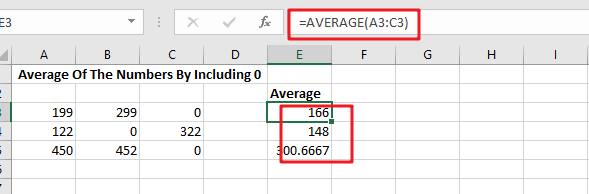### General Formula

Use the formula below to calculate the average of a set of numbers in Excel:

`=AVERAGE(range)`

Explanations for Syntax:

•  `AVERAGE`: In Excel, the AVERAGE Function can calculate the arithmetic mean of a set of numbers.
• `Range`: This is the input value from your worksheet.
• `Comma symbol (,):` It acts as a separator that helps to separate a list of values.
• `Parenthesis ()`: The main Function of this symbol is to group the elements.

### Explanation

Use the AVERAGE function to calculate the average of a set of numbers.

In the example, the formula in E3 is:

`=AVERAGE(A3:C3)`which is then copied down in the table.

AVERAGE is a built-in function in MS Excel in which you supply a range of cells to average, and this function returns the result. In cases where cells are not adjacent, you can also provide individual arguments to the Function.

The AVERAGE function automatically ignores all the blank text and cells, but zero values are included.

## Average Of The Numbers By Ignoring 0

### General Formula

The formula below will assist you in calculating the average of a number that excludes zero:

`=AVERAGEIF(range,"<>0")`

Explanations for Syntax:

AVERAGEIF: This Function returns the average of a set of input values that satisfy a single condition or criteria. Learn more about the AVERAGEIF Function.

Range: This is the input value from your worksheet.

Comma symbol (,): It acts as a separator that helps to separate a list of values.

Parenthesis (): The main Function of this symbol is to group the elements.

Operator (>): The supplied criteria is “>0,” which means “not equal to zero.”

### Explanation

The AVERAGEIF function computes the average of a set of numbers while excluding or ignoring zero values. In the example, the formula in E10 is:

`=AVERAGEIF(A10:C10,"<>0")`

###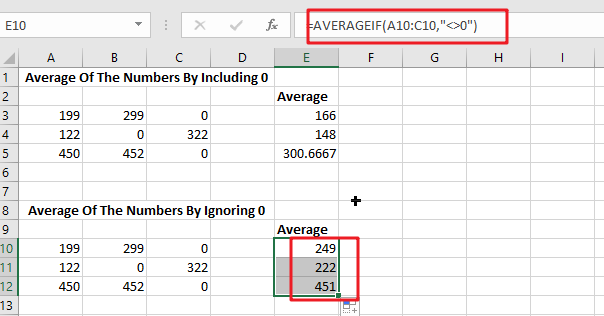The formula in E3 in the example is based on the AVERAGE Function:

`=AVERAGE(A3:C3)`

Because (129+299+0)/ 3 = 166, the answer is 166.

To remove the zero from the calculated average, the formula in E10 employs the AVERAGEIF function, as shown below:

`=AVERAGEIF(A10:C10,"<>0") / returns 249`

The provided criterion is “>0,” which means “not equal to zero”.

### Data with no values

Because the AVERAGE, AVERAGEIF, and AVERAGEIFS functions all ignore blank cells (and cells with text values), there isn’t any need to provide criteria to filter out empty cells.

## Average Of The Top 3 Numbers

### General Formula

The formula below will assist you in calculating the average of the top 3 numbers:

`=AVERAGE(LARGE(range,{1,2,3}))`

Explanations for Syntax:

• `AVERAGE:` In Excel, the AVERAGE Function can calculate the arithmetic mean of a set of numbers.
• `Range: `This is the input value from your worksheet.
• `Comma symbol (,)`: It acts as a separator that helps to separate a list of values.
• `Parenthesis ()`: The main Function of this symbol is to group the elements.
• `LARGE: `This Function returns the Nth largest value from the given range of data. Learn more about the LARGE Function.
• `Values:` This is the range of cells that contain values.

### Explanation

To average the top three values in a data set, use a formula based on the LARGE and AVERAGE functions. In this example, the formula in G18 is mentioned below:

`=AVERAGE(LARGE(A18:E18,{1,2,3}))`

###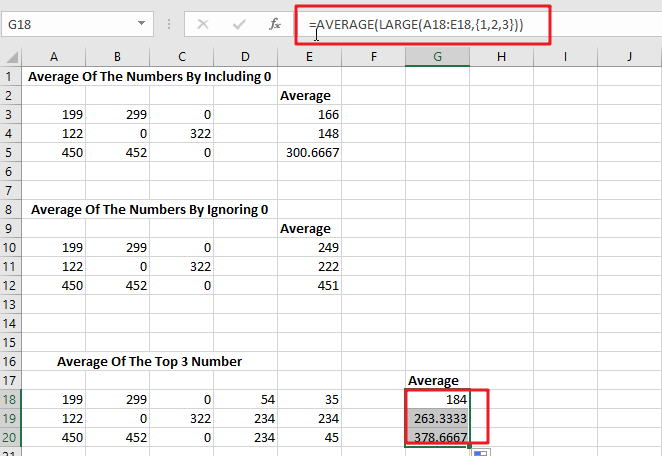The LARGE function is used to find the top nth value in a set of numbers. For example, the LARGE(A18:E18,{1,2,3})will return the highest value, LARGE(A18:E18,2) will return the second-highest value, and so on:

```=LARGE(A18:E18,1)/ First largest value
=LARGE(A18:E18,2)/ 2nd largest value
=LARGE(A18:E18,3)/ 2nd largest value```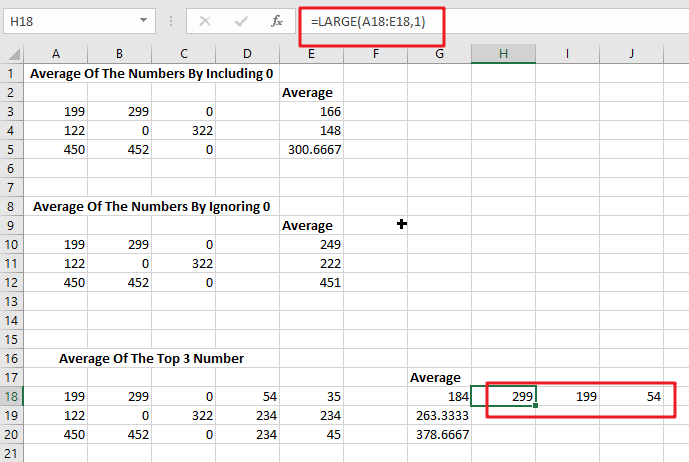In this case, we are requesting multiple values by passing an array constant 1,2,3 into LARGE as the second argument. This causes LARGE to return an array of the top three values.

`=LARGE(A18:E18,{1,2,3})`

returns an array similar to this:

`{299,199,54}`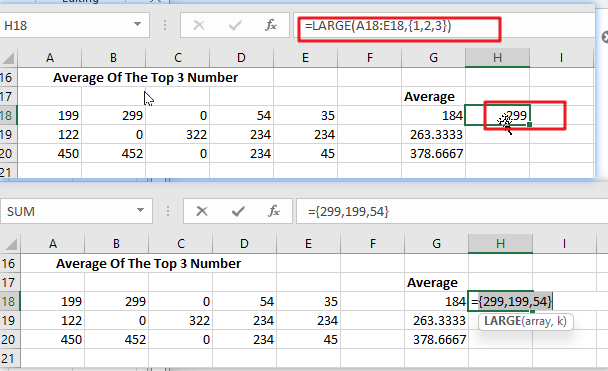This array is passed directly to the AVERAGE function:

`=AVERAGE(299,199,54) / returns 184`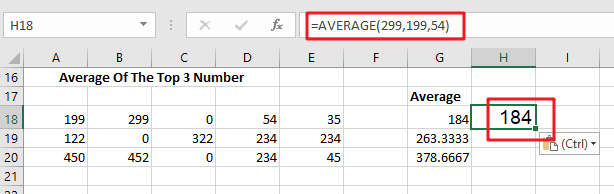The AVERAGE Function then returns the average of these values.

Because the AVERAGE Function can handle arrays natively, there is no need to enter this formula with control + shift + enter.

### Related Functions

• Excel AVERAGE function
The Excel AVERAGE function returns the average of the numbers that you provided.The syntax of the AVERAGE function is as below:=AVERAGE (number1,[number2],…)….
• Excel AVERAGEIF function
The Excel AVERAGEAIF function returns the average of all numbers in a range of cells that meet a given criteria.The syntax of the AVERAGEIF function is as below:= AVERAGEIF (range, criteria, [average_range])….
• Excel LARGE function
The Excel LARGE function returns the largest numeric value from the numbers that you provided. Or returns the largest value in the array. The syntax of the LARGE function is as below:= LARGE (array,nth)…

## Extract or Filter on The Top N Values with Criteria

Suppose that you are in a situation where you need to filter out the top n values from the list having few values with specific criteria, or you can say that with a particular condition, and I am also pretty sure about it that you would definitely choose to do it manually, which is also a great choice when you have only a few values in a list, and you want to filter out the top n values with a certain criteria (condition).

But if you are dealing with multiple values in the list and you want to filter out the top n values with a specific criteria (condition), then in such a situation doing these tasks manually would be a foolish act because doing it manually, there are 90% chances that you would 100% get tired of it and can’t complete your task at the right time.

But don’t be worry about it because after carefully reading this article filtering out the top n values with the particular criteria (condition) from the list containing multiple values would become a piece of cake for you.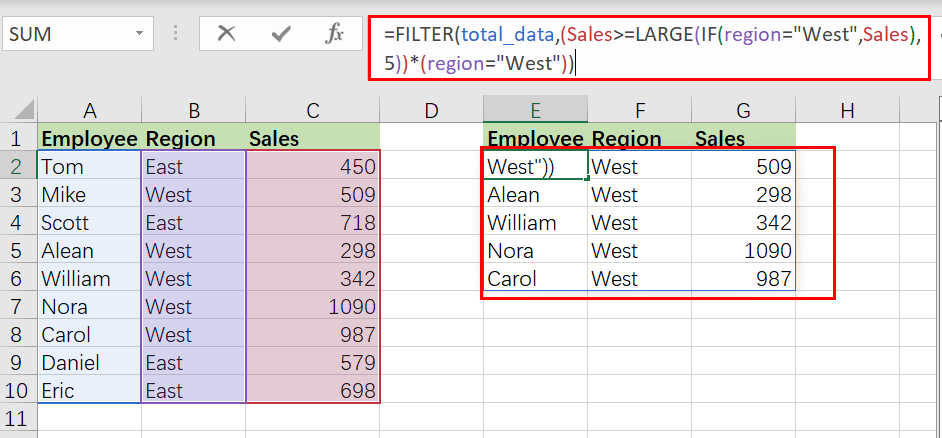So let’s dive into the article to take you out of this fix.

## General Formula

The Following formula would help you Filter on top n values with the specific criteria (condition) in MS Excel:

`=FILTER(total_data,(condition_range>=LARGE(IF(condition),n))*( condition))`

## Syntax Explanations

Before going into the explanation of the formula for getting the work done efficiently, we must understand each syntax which would make it easy for us that how each syntax contributes to filtering out the top n values in MS Excel.

• `Filter`: This function contributes to narrowing down or filtering out a range of data on the user-defined criteria.
• `total_data`: In your worksheet, it represents the input ranges.
• `Comma symbol` (,): In Excel, this comma symbol acts as a separator that helps to separate a list of values.
• `condition_range`: In Excel, the range is nothing but the difference between highest and lowest values
• `Parenthesis` (): The core purpose of this Parenthesis symbol is to group the elements and separate them from the rest of the elements.
• `Greater than Equal To Symbol` (>=): Greater than Equal To Symbol (>=) is used to identify the values which are either greater than or equal to.
• `LARGE`: Large function is used to get the higher arguments.
• `IF`( ): IF function is the most popular function in excel, which checks whether the condition is met.
• `Criteria`: This means the condition to which the user desires to get the results according to it.

## Let’s See How This Formula Works

For instance, you got a task in which there is a table where you have candidates of some regions (i.e., region East and region West ) and which are assigned to a particular sales, now you want to filter out the top 5 candidates with the higher sales along with the criteria that those top 5 candidates must be from the region West, now let’s analyze that how to to write the formula and how this formula would do it.

As to filter on the top n values with criteria, we would write the formula according to the given list like:

`=FILTER(total_data,( Sales >=LARGE(IF(region="West", Sales),5))*( region =" West"))`Here the` region`` (B2:B10)`, `Sales`` (C2:C10)`, and `total_data`` (A2:C10)` are the named ranges in the above example.

The FILTER function is used in this formula to obtain data based on a logical test built using the LARGE and IF functions. The top five sales in `region West` are the outcome.

The FILTER function uses the include parameter to apply criteria. Criteria in this example are built using boolean logic, as seen below:

`=(Sales >=LARGE(IF(region="West", Sales),5))*( region =" West"))`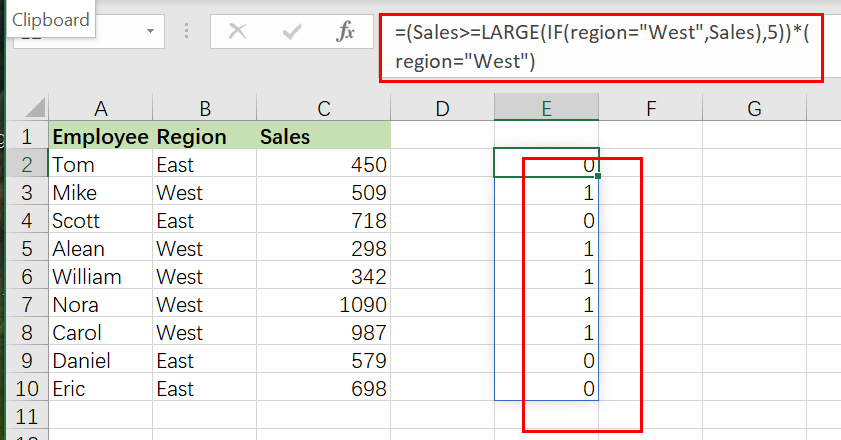The expression’s left side seeks sales greater than or equal to the fifth-highest sale in region West:

`=IF(region="West", Sales)`

The IF function ensures that LARGE only works with region West sales. Because we have a total of 9 sales, IF produces an array with 9 outcomes, as seen below:

`{FALSE;509;FALSE;298;342;1090;987;FALSE;FALSE}`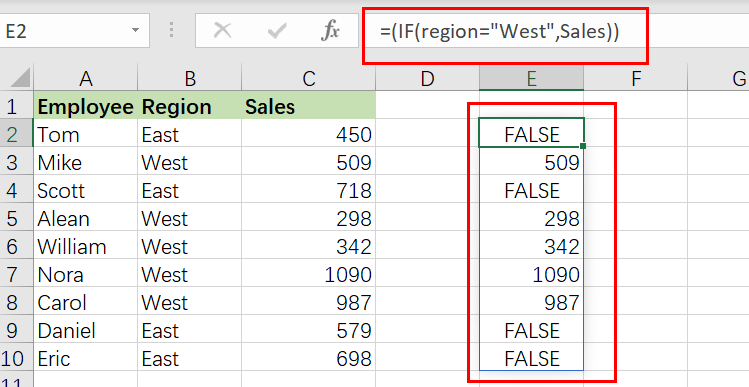It’s worth noting that the only sales that survive the procedure are from region West. All other results are FALSE. As the array parameter, this array is returned straight to LARGE:

`=LARGE({FALSE;509;FALSE;298;342;1090;987;FALSE;FALSE},5)`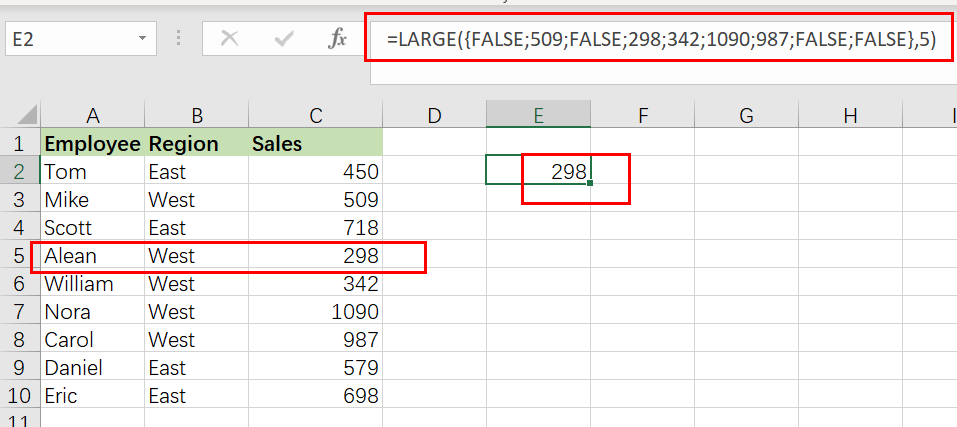The FALSE values are ignored by LARGE, which yields the Fifth-highest sales, `298`.

We can now reduce the formula to:

`=FILTER(total_data ,(Sales>=298)*( region =" West"))`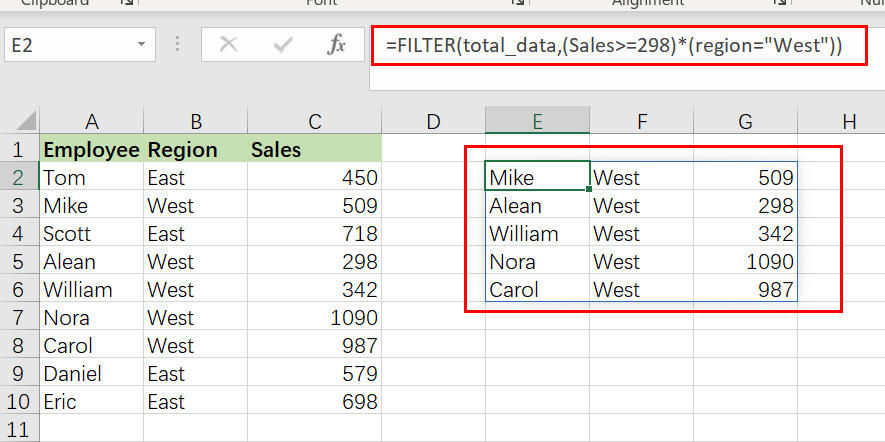Finally, FILTER retrieves results in the `E2:G6 range`.

## Sort results by Sales Value Using Sort Function

FILTER will return matched entries in the order they occur in the supplied data by default. To sort results by sales in decreasing order, nest the original FILTER formula within the SORT function as follows:

`=SORT(FILTER(total_data,( Sales >=LARGE(IF(region="West", Sales),5))*( region =" West")),3,-1)`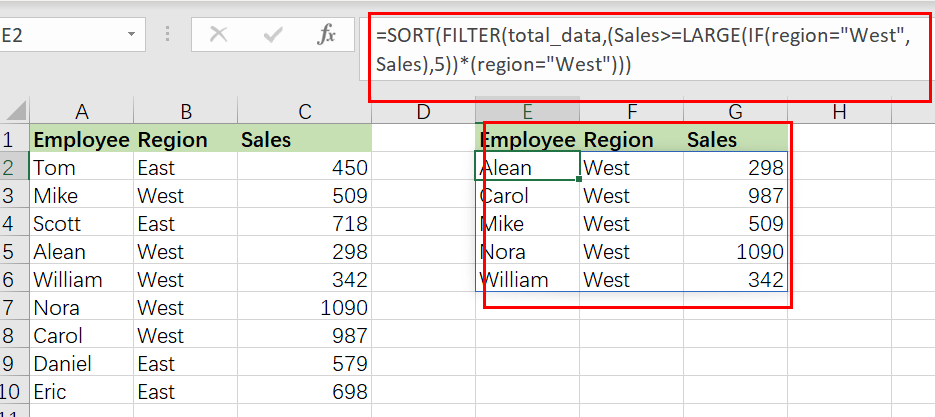In this case, FILTER passes the results to the SORT function as an array parameter. The sort index is set to 3(sort results based on Sales values),while the sort order is set to -1 (descending order).

### Related Functions

• Excel LARGE function
The Excel LARGE function returns the largest numeric value from the numbers that you provided. Or returns the largest value in the array. The syntax of the LARGE function is as below:= LARGE (array,nth)…
• Excel IF function
The Excel IF function perform a logical test to return one value if the condition is TRUE and return another value if the condition is FALSE. The IF function is a build-in function in Microsoft Excel and it is categorized as a Logical Function.The syntax of the IF function is as below:= IF (condition, [true_value], [false_value])….
• Excel Filter function
The FILTER function extracts matched records from a collection of data using one or more logical checks. The include argument specifies logical tests, which might encompass a wide variety of formula conditions.==FILTER(array,include,[if empty])…
• Excel Sort function
The SORT function in Excel sorts the contents of an array or range alphabetically or numerically by columns or rows.The syntax:=SORT(array, [sort index],) …

## Average of Top N Values in Excel

AVERAGE function is one of the most popular functions in Excel. Apply AVERAGE together with some other functions, we can calculate average properly based on different situations.

In this article, we will introduce you the way to calculate average of the top N numbers from a range. To approach our goal, except the main AVERAGE function, we also apply LARGE function.

## EXAMPLE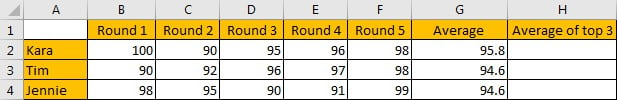In this case, we want to calculate the average of the top three scores from 5-rounds competitions for each student. To calculate the average of top N values, we need the help of LARGE function.

## SOLUTION

To create a formula to get average in this case, we need to know:

1) Find out the top three values by LARGE function.

2) Calculate average of the top three values by AVERAGE function.

The general formula is =AVERAGE(LARGE(Range,{1,2,…,N})).

## FORMULA with AVERAGE & OTHER FUNCTIONS

In C2, we input the formula =AVERAGE(LARGE(B2:F2,{1,2,3})). After typing, press Enter, then average “98” of the top three scores is returned.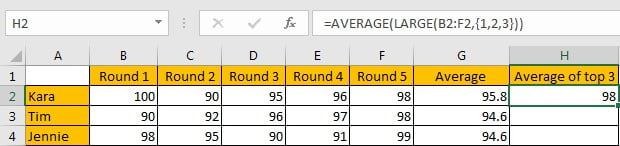For Kara, five scores are saved in range B2:F2, the top three values are 100, 96 and 98, so the average is (100+96+98)/3=98, the formula returns correct result.

## FUNCTION INTRODUCTION

The main function in this formula is “AVERAGE”, it returns the average of the top three values by our demands; “LARGE” is used for searching for the top three values among all given values.

a. LARGE function returns the Kth largest number from a given set of numbers or a range reference.

Syntax:

`=LARGE(array,k)`

Example: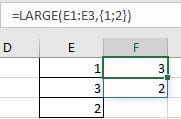//If we input 2 for, only the second top value is returned;

//If we want to returning more than one top value, we can set an array constant as the function’s second argument;

//If we input {1;2} for k, 1 and 2 are separated by “;”, the top two values are saved in the same column;

//If we input {1,2} for k, 1 and 2 are separated by “,”, the top two values are saved in the same row.

b. AVERAGE function returns the average of numbers from a given range reference.

Syntax:

`=AVERAGE(number1, [number2], …)`

Example: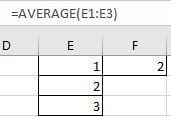//For arguments “number1, number2,…”, they can be a set of numbers, or an array of numbers like {1;2;3}.

FORMULA EXPLANATION

`=AVERAGE(LARGE(B2:F2,{1,2,3}))`

//This formula contains 2 functions, we explain functions from inside to outside.

a. For LARGE(B2:F2,{1,2,3}), range B2:F2 provides all scores from 5 rounds competitions; array constant {1,2,3} is the second argument “k” value, it determines the count of top numbers returned by LARGE.

In this case, B2:F2 is {}, LARGE returns below array:

`LARGE({100,90,95,96,98},{1,2,3}) -> {100,98,96}`

b. Array {100,98,96} from last step goes into AVERAGE calculation now.

=AVERAGE({100,98,96})

So, we get 98 properly after above two steps.

### Related Functions

• Excel AVERAGE function
The Excel AVERAGE function returns the average of the numbers that you provided.The syntax of the AVERAGE function is as below:=AVERAGE (number1,[number2],…)….
• Excel LARGE function
The Excel LARGE function returns the largest numeric value from the numbers that you provided. Or returns the largest value in the array. The syntax of the LARGE function is as below:= LARGE (array,nth)…

## Average the Last N Numeric Values in Excel

AVERAGE function is one of the most popular functions in Excel. Apply AVERAGE together with some other functions, we can calculate average simply for some complex situations.

In this article, we will introduce you to calculate average of the last N numbers from a range contains both numeric values and non-numeric values.

## EXAMPLE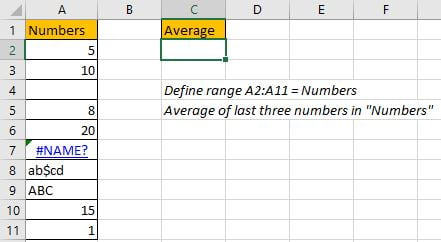In this case, we want to calculate the average of the last 3 numeric values in “Numbers” column. As non-numeric value “ABC” exists in last three cells, so we cannot directly apply AVERAGE(A9:A11) directly. To calculate average ignoring invalid values, we need the help of other functions.

In this article, to approach our goal, except the main AVERAGE function, we also apply ROW, ISNUMBER, IF, LARGE, LOOKUP functions.

## SOLUTION

To create a formula to get average in this case, we need to know:

1) Distinguish numbers and non-numeric values from range “Numbers”. Ignore non-numeric values in calculation.

2) Find out the last three cells with numbers in “Numbers”.

3) Find out last three values through searching for the last three positions.

## FORMULA with AVERAGE & OTHER FUNCTIONS

In C2, we input the formula

`=AVERAGE(LOOKUP(LARGE(IF(ISNUMBER(Numbers),ROW(Numbers)),{1,2,3}), ROW(Numbers), Numbers)).`

After typing, press Enter, average of the last three numbers is 12.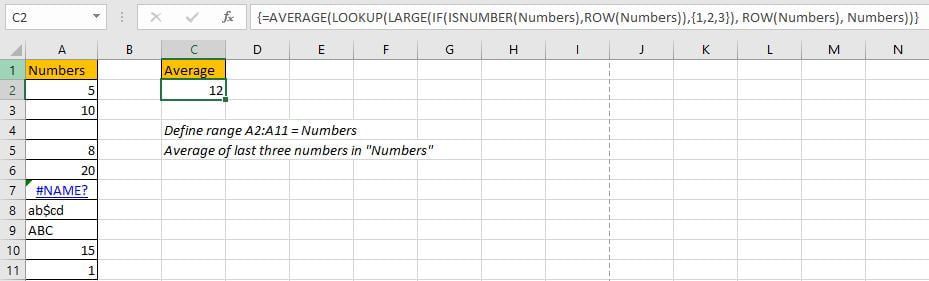In “Numbers” list, the last three values are 20, 15 and 1, the average is (20+15+1)/3=12, so the formula returned value is correct. The last three values are found out properly.

## FUNCTION INTRODUCTION

The main function in this formula is “AVERAGE”, it can return the average of last three values; Others are supported to find out numbers (ignoring non-numeric values), mark row numbers for the last three numbers, and the through searching row numbers to return corresponding numbers.

1. ROW function returns row number for a given range reference.

Syntax:

`=ROW(reference)`

Example. ROW(A1:A3) returns row numbers of range A1:A3, so we get {1;2;3}.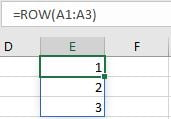2. ISNUMBER function returns True (for numeric values) or False (for strings, errors) based on value is a numeric value or not. For blank cells, it returns False.

Syntax:

`=ISNUMBER(value)`

Example. You can input a value, a cell reference, or a range reference for “value”.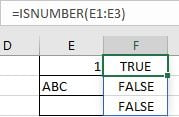3. IF function returns “true value” or “false value” based on the result of provided logical comparison. It is one of the most popular function in Excel.

Syntax:

`=IF(logical_test,[value_if_true],[value_if_false])`

Example, =IF(ISNUMBER(E1:E3),”Yes”,”No”).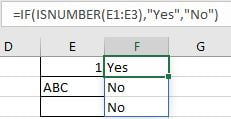4. LARGE function returns the Kth largest number from a given set of numbers or a range reference.

Syntax:

`=LARGE(array,k)`

Example, =LARGE(E1:E3,{1;2}), an array {3;2} is returned. If you set k=2, only 2 is returned.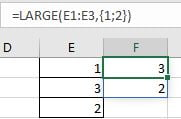5. LOOKUP function can through searching for a row or column to return the corresponding value in the same position but in the second row or column. It has two different syntaxes, in this case, it is for vector:

Syntax:

`=LOOKUP(lookup_value, lookup_vector, [result_vector])`

Example, =LOOKUP(H2,E2:E3,F2:F3), apply lookup to find out name for id1.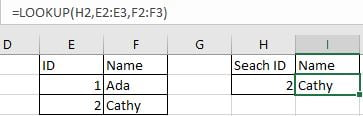6. AVERAGE function returns the average of numbers from a given range reference.

Syntax:

`=AVERAGE(number1, [number2], …)`

Example. For arguments “number1, number2,…”, they can be a set of numbers, or an array of numbers like {1;2;3}.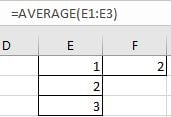## FORMULA EXPLANATION

`=AVERAGE(LOOKUP(LARGE(IF(ISNUMBER(Numbers),ROW(Numbers)),{1,2,3}), ROW(Numbers), Numbers))`

This formula contains 6 functions, we explain functions from inside to outside.

1. For ISNUMBER(Numbers), “Numbers” is A2:A11, so ISNUMBER function check values in each cell in this range and returns “True” for numeric values and “False” for non-numeric values or blank. ISNUMBER(Numbers) returns below array:

`{TRUE;TRUE;FALSE;TRUE;TRUE;FALSE;FALSE;FALSE;TRUE;TRUE}`

2. ROW(Numbers)

` returns row numbers for range A2:A11.`
`{2;3;4;5;6;7;8;9;10;11}`

3. With the help of ISNUMBER and ROW functions, IF function returns row numbers for cells with numeric values.

`IF({TRUE;TRUE;FALSE;TRUE;TRUE;FALSE;FALSE;FALSE;TRUE;TRUE},{2;3;4;5;6;7;8;9;10;11})`

Based on logical test result, IF returns row number for “true value” and keeps “False” for “false value” as [value_if_false] is omitted. Then we get below array from IF function:

`{2;3;FALSE;5;6;FALSE;FALSE;FALSE;10;11}`

4. Now above array returned from IF function participates into LARGE function calculation.

`LARGE({2;3;FALSE;5;6;FALSE;FALSE;FALSE;10;11},{1,2,3})`

K is an array {1,2,3}, so LARGE function returns the largest three values from array {2;3;FALSE;5;6;FALSE;FALSE;FALSE;10;11}. In this step, LARGE function returns the last three row numbers for cells with numbers.

`{11,10,6}`

5. In step#4, we get the last three row numbers properly. Then we can apply LOOKUP function to lookup corresponding values in row 11, row 10 and row 6 from range “Numbers”.

Row(Numbers) is applied twice in this formula, the first one is “value_if_true” for IF function, the second one is used as  “lookup_vector” for LARGE function.

`LOOKUP({11,10,6}, ROW(Numbers), Numbers)=LOOKUP({11,10,6}, {2;3;4;5;6;7;8;9;10;11}, {5;10;0;8;20;#NAME?;"ab\$cd";"ABC";15;1})`

In this step, LOOKUP function returns proper values from A2:A11 after searching for row numbers 11, 10 and 6. So we get below array at last:

`{1,15,20}`

6. After above all steps, we get =AVERAGE({1,15,20}). So, the returned value is (1+15+20)/3=12.

### Related Functions

• Excel ROW function
The Excel ROW function returns the row number of a cell reference.The ROW function is a build-in function in Microsoft Excel and it is categorized as a Lookup and Reference Function.The syntax of the ROW function is as below:= ROW ([reference])….
• Excel IF function
The Excel IF function perform a logical test to return one value if the condition is TRUE and return another value if the condition is FALSE. The IF function is a build-in function in Microsoft Excel and it is categorized as a Logical Function.The syntax of the IF function is as below:= IF (condition, [true_value],
• Excel ISNUMBER function
The Excel ISNUMBER function returns TRUE if the value in a cell is a numeric value, otherwise it will return FALSE.The syntax of the ISNUMBER function is as below:= ISNUMBER (value)…
• Excel LOOKUP function
The Excel LOOKUP function will search a value in a vector or array.The syntax of the LOOKUP function is as below:= LOOKUP (lookup_value, lookup_vector, [result_vector])…
• Excel AVERAGE function
The Excel AVERAGE function returns the average of the numbers that you provided.The syntax of the AVERAGE function is as below:=AVERAGE (number1,[number2],…)….
• Excel LARGE function
The Excel LARGE function returns the largest numeric value from the numbers that you provided. Or returns the largest value in the array. The syntax of the LARGE function is as below:= LARGE (array,nth)…

## How To Sum the Largest N Values in Excel

Sometimes we may want to sum the largest N numbers or top N numbers in a range. In this article, we will show you the method of “SUM the Largest N Numbers” by a simple formula which consist of SUMPRODUCT and LARGE functions. LARGE can return largest values based on criteria, SUMPRODUCT can sum up these largest values.

Through a simple instance, we will introduce you the syntax, argument and the usage of SUMPRODUCT and LARGE functions. We will let you know how the formula works step by step clearly. After reading the article, you may know that in which situations we can choose SUMPRODUCT function to sum data.

## EXAMPLE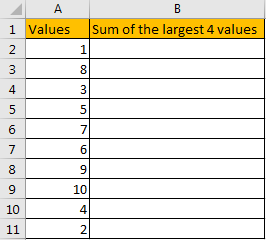In the ‘Values’ column, there are 10 values from ‘1’ to ‘10’ in improper order. Now we want to sum the largest 4 values from this list, how can we get the correct result?

Actually, we can sort these numbers from the largest to the smallest by ‘Sort Z-A’ in excel.

Click ‘Data’ tab, under ‘Sort & Filter’ section click ‘Sort Z to A’ icon.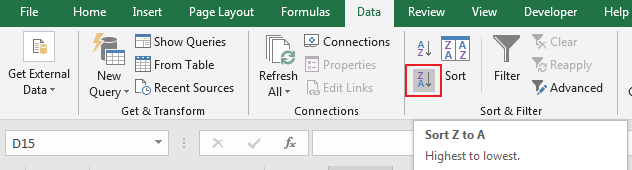Then you can find numbers are ordered properly.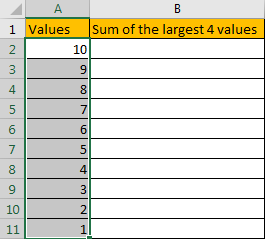Then you can enter SUM function in B2 and select the first four numbers ->cell reference A2:A5 in the list.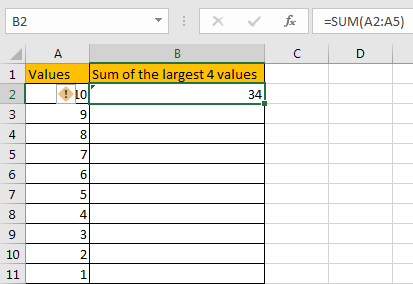Then you can get the sum of the largest four values. It is 34.

In this way, we can get result correctly. But we can also get it conveniently and correctly by just enter a formula. As we want to sum the largest four numbers from range A2:A11, we can find the largest N number or numbers by LARGE function actually, and then use SUMPRODUCT function to sum array directly.

## FORMULA – APPLY SUMPRODUCT FUNCTION

Step 1: In B2, enter the formula

` =SUMPRODUCT(LARGE(A2:A11,{1,2,3,4})).`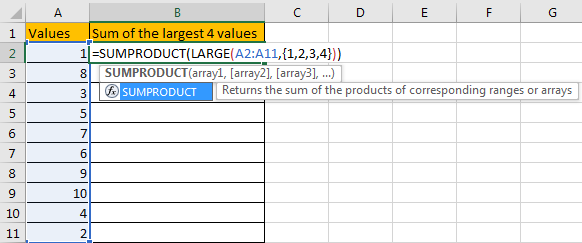Step 2: Press Enter after typing the formula.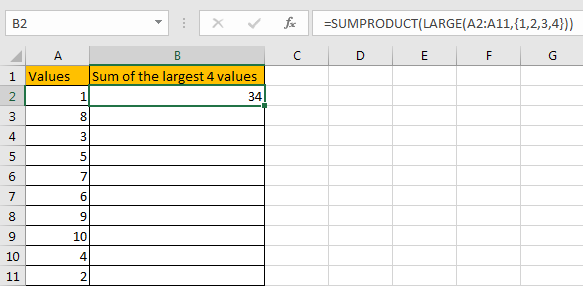We can see that this time we also get correct result 34. The formula works correctly.

## FUNCTION INTRODUCTION

SUMPRODUCT function can be seen as SUM+PRODUCT.

For SUMPRODUCT function, the syntax is:

`=SUMPRODUCT(array1,array2,array3, ...)`

For example, enter =SUMPRODUCT({1,2},{2,3}) in any cell, the we can get value 8, it equals to 1*2+2*3=8. You can also enter =SUMPRODUCT({1,2}*{2,3}), you can get the same result. The argument array can be a real array {1,2,3} for example or a range reference like A2:A7.

If there is only array in the formula, SUMPRODUCT will sum the numbers in the array.

SUMPRODUCT function allows entering texts, logical operators and expressions like (product=cap), texts should be enclosed into ().

LARGE function can return the Nth largest value in a range.

For LARGE function, the syntax is:

`=LARGE(array,k)`

If k is a number, it returns the Kth largest value in the range; if k is an array like {1,2,3}, it returns the largest three values in the range. If k is an array, but the numbers are in inconsecutive sequence for example k={1,2,4}, then LARGE function will return the 1st, 2nd, 4th largest values in the range.

## ARGUMENTS EXPLAINATION

In this formula =SUMPRODUCT(LARGE(A2:A11,{1,2,3,4})), we applied two functions.

For LARGE function, range is A2:A11, k is an array {1,2,3,4}.

Select range A2:A11 in formula bar, press F9 to convert cell reference to real values, numbers in ‘Values’ column are expanded in an array.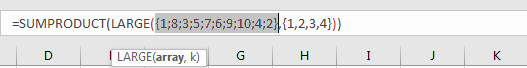For SUMPRODUCT function, there is only one array, it is the result of formula LARGE({1;8;3;5;7;6;9;10;4;2},{1,2,3,4}).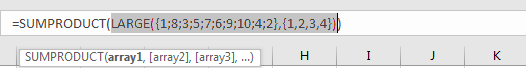## HOW THIS FORMULA WORKS

After explaining argument in the formula, the formula is converted to =SUMPRODUCT(LARGE({1;8;3;5;7;6;9;10;4;2},{1,2,3,4})) in the formula bar. Now we will show you how the formula works with the two functions.

For LARGE({1;8;3;5;7;6;9;10;4;2},{1,2,3,4}), refer to k value {1,2,3,4}, we find out the largest 4 values in the array {1;8;3;5;7;6;9;10;4;2}.

Select LARGE({1;8;3;5;7;6;9;10;4;2},{1,2,3,4}) in the formula bar, press F9. We can get the result {10,9,8,7}. Numbers are ordered from the largest to the smallest.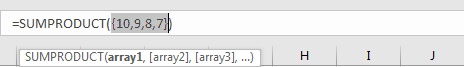Obviously, the final result is 10+9+8+7=34. Select =SUMPRODUCT({10,9,8,7}) in the formula bar, press F9, 34 is displayed in formula bar.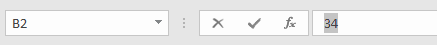1. In this instance, there are only 10 numbers in the range, and we only want to sum the largest 4 numbers. If there are a large amount of numbers, and sum the largest 100 or more numbers in the range, do we need to enter {1,2,3,4,…,100} in LARGE function? Obviously, it is very complex and troublesome. So, we can solve this problem by the help of ROW and INDIRECT

For example, if we want to sum the largest 9 numbers in the same range, we can enter the follow formula in Cell B2:

`=SUMPRODUCT(LARGE(A2:A11,ROW(INDIRECT("1:9"))))`

Then we can get correct value 54.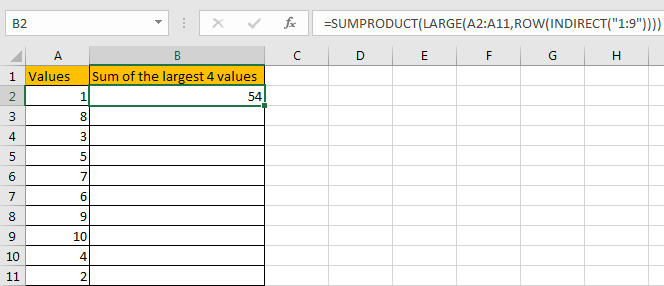In this case, INDIRECT function can return a valid reference based on entered texts. With the help of ROW, ROW(INDIRECT(“1:9”)) can convert “1:9” to {1,2,3,4,5,6,7,8,9}.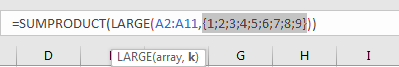2. If N number is saved in another cell, we can concentrate N inside INDIRECT function. Enter the follow formula in Cell d2 this time:

`=SUMPRODUCT(LARGE(A2:A11,ROW(INDIRECT("1:"&D1))))`

See example below: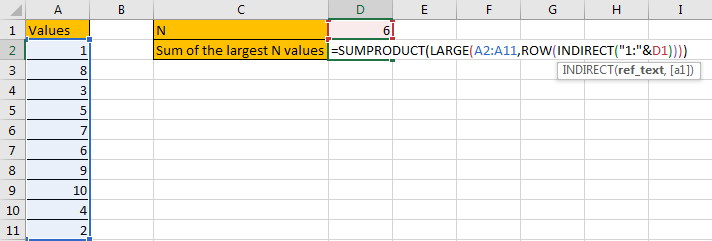In this case, when changing the number in D1, result in D2 will be automatically updated. We don’t need to adjust the formula or cell reference. See example below.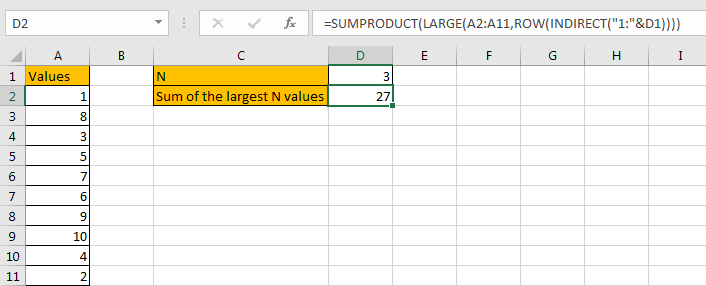3. In this case we can also use SUM function to replace SUMPRODUCT function. But SUMPRODUCT function can handle arrays directly without entering Ctrl + Shift + Enter, so we often apply SUMPRODUCT function in similar situations.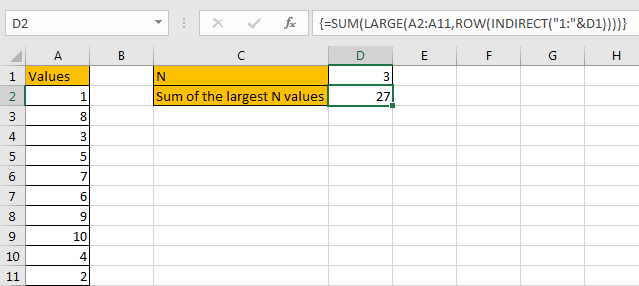SUMMARY

1. SUMPRODUCT function can handle multiple arrays, and it sum up the products.

2. SUMPRODUCT function allows user defined range, wildcards, logical operators, expressions.

3. LARGE function can return the Nth or first Nth largest value in a range.

4. ROW(INDIRECT(“1:N”)) can return a set of numeric numbers in an array.

5. If N is in another cell, use “&” to concentrate them inside INDIRECT, for example ROW(INDIRECT(“1:”&B2)).

### Related Functions

• Excel SUMPRODUCT function
The Excel SUMPRODUCT function multiplies corresponding components in the given one or more arrays or ranges, and returns the sum of those products. The syntax of the SUMPRODUCT function is as below:= SUMPRODUCT (array1,[array2],…)…
• Excel ROW function
The Excel ROW function returns the row number of a cell reference.The ROW function is a build-in function in Microsoft Excel and it is categorized as a Lookup and Reference Function.The syntax of the ROW function is as below:= ROW ([reference])….
• Excel LARGE function
The Excel LARGE function returns the largest numeric value from the numbers that you provided. Or returns the largest value in the array. The syntax of the LARGE function is as below:= LARGE (array,nth)…
• Excel INDIRECT function
The Excel INDIRECT function returns the cell reference based on a text string, such as: type the text string “A2” in B1 cell, it just a text string, so you can use INDIRECT function to convert text string as cell reference….
• Excel SUM function
The Excel SUM function will adds all numbers in a range of cells and returns the sum of these values. You can add individual values, cell references or ranges in excel.The syntax of the SUM function is as below:= SUM(number1,[number2],…)

## How to Extract Number from Text String in Excel

This post will guide you how to extract number from a given test string in Excel. How do I extract all numbers from string using a formula in Excel. How to get all number from a given test string using user defined function in Excel.

Assuming that you have a list of data in range B1:B5, in which contain text string values, and you want to eextract all numbers from each cell in which contain letters and numbers. In other words, you wish to remove all letters from those text strings. And this post will show you two methods to accomplish it.

## Extract Number from String with Formula

If you want to extract numbers from a range of cells that contain text string values, you need to use an array formula based on the SUM function, MID function, LARGE function, INDEX function, ISNUBER function and the ROW function to extract all numbers from one cell that contain text string values. Like this:

`=SUM(MID(0&B1,LARGE(INDEX(ISNUMBER(--MID(B1,ROW(\$1:\$99),1))*ROW(\$1:\$99),),ROW(\$1:\$99))+1,1)*10^ROW(\$1:\$99)/10)`

You need to type this formula into a blank cell and press Ctrl + Shift +Enter key on your keyboard to change the current common formula as Array formula.  Then drag the AutoFill handle down to other cells.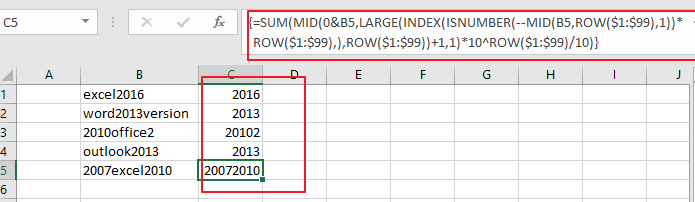Note: B1 is the cell that you want to extract numbers from.

## Extract Number from String with User Defined Function

You can also use an VBA code to define a User defined function to accomplish the same result of extracting all numbers from text string in Excel. Just do the following steps:

Step1# open your excel workbook and then click on “Visual Basic” command under DEVELOPER Tab, or just press “ALT+F11” shortcut.Step2#  then the “Visual Basic Editor” window will appear.

Step3# click “Insert” ->”Module” to create a new module.Step4# paste the below VBA code into the code window. Then clicking “Save” button.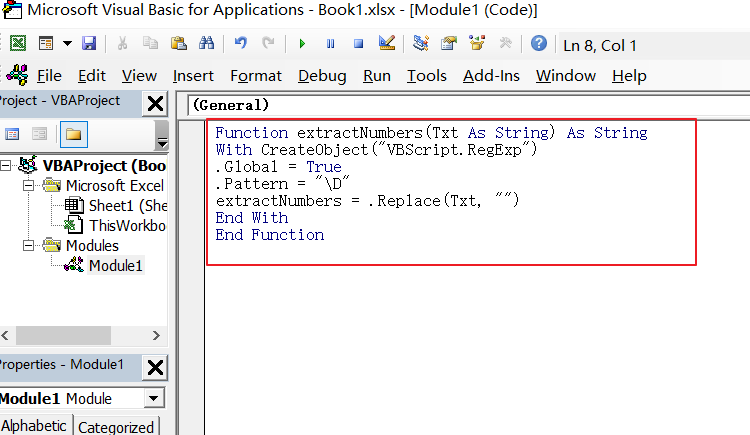[vb]
Function extractNumbers(Txt As String) As String
With CreateObject("VBScript.RegExp")
.Global = True
.Pattern = "\D"
extractNumbers = .Replace(Txt, "")
End With
End Function
[/vb]

#5 back to the current worksheet, then type the following formula in a blank cell, and then press Enter key. And drag the AutoFill Handle over to other cells.

=extractNumbers(B1)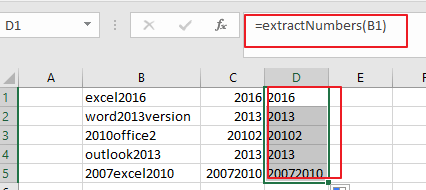### Related Functions

• Excel SUM function
The Excel SUM function will adds all numbers in a range of cells and returns the sum of these values. You can add individual values, cell references or ranges in excel.The syntax of the SUM function is as below:= SUM(number1,[number2],…)…
• Excel INDEX function
The Excel INDEX function returns a value from a table based on the index (row number and column number)The INDEX function is a build-in function in Microsoft Excel and it is categorized as a Lookup and Reference Function.The syntax of the INDEX function is as below:= INDEX (array, row_num,[column_num])…
• Excel ISNUMBER function
The Excel ISNUMBER function returns TRUE if the value in a cell is a numeric value, otherwise it will return FALSE.The syntax of the ISNUMBER function is as below:= ISNUMBER (value)…
• Excel ROW function
The Excel ROW function returns the row number of a cell reference.The ROW function is a build-in function in Microsoft Excel and it is categorized as a Lookup and Reference Function.The syntax of the ROW function is as below:= ROW ([reference])….
• Excel MID function
The Excel MID function returns a substring from a text string at the position that you specify.The syntax of the MID function is as below:= MID (text, start_num, num_chars)…
• Excel LARGE function
The Excel LARGE function returns the largest numeric value from the numbers that you provided. Or returns the largest value in the array. The syntax of the LARGE function is as below:= LARGE (array,nth)…

## Find the Earliest and Latest Date in a Range of Dates in Excel

This post will guide you how to find the earliest date in a range of dates in Excel. How do I get the earliest and latest date in a range with a formula in Excel. How to find the highest and lowest date in a range of dates in Excel.

Assuming that you have a list of data in a range A1:C3 that contain dates, and you want to get the earliest date in these dates, how to achieve it. You can use a formula based on the MIN function or the SMALL function to get the earliest date. And if you want to get the latest date in those range of cells, you can use a formula based on the MAX function or the LARGE function.

## Find Earliest (Lowest) Date

If you want to find the earliest date in the range of cells A1:C3, just type the following formula into a blank cell, and then press Enter key.

`=MIN(A1:C3)`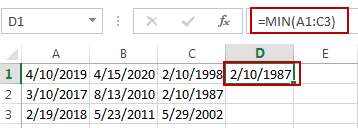Or

`=SMALL(A1:C3,1)`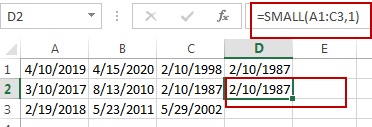## Find Latest Date

If you want to get the latest date in a range of cells, you can use the following formula, and type it into a blank cell, and then press Enter key.

`=MAX(A1:C3)`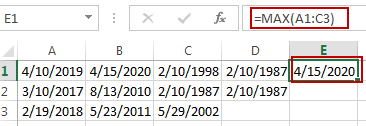Or

`=LARGE(A1:C3,1)`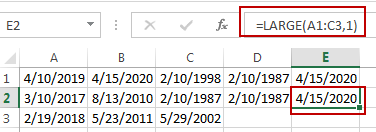### Related Functions

• Excel MIN function
The Excel MIN function returns the smallest numeric value from the numbers that you provided. Or returns the smallest value in the array.The MIN function is a build-in function in Microsoft Excel and it is categorized as a Statistical Function.The syntax of the MIN function is as below:= MIN(num1,[num2,…numn])….
• Excel MAX function
The Excel MAX function returns the largest numeric value from the numbers that you provided. Or returns the largest value in the array.= MAX(num1,[num2,…numn])…
• Excel LARGE function
The Excel LARGE function returns the largest numeric value from the numbers that you provided. Or returns the largest value in the array. The syntax of the LARGE function is as below:= LARGE (array,nth)…
• Excel SMALL function
The Excel SMALL function returns the smallest numeric value from the numbers that you provided. Or returns the smallest value in the array.The syntax of the SMALL function is as below:=SMALL(array,nth) …

## Find Closest Value or Nearest Value in a Range in Excel

This post will guide you how to find the closest value or nearest value in a range of cells or in a column in Excel. How do I find the closest match in a range to a given lookup value in Excel. How to find the closest larger and closest smaller values from a range of cells in excel.

## Find Closest Value or Nearest Value in a Range

Assuming that you have a list of numbers in range of cells B1:B6, and you have a lookup value in Cell C1, and you want to find the nearest value that matches the lookup value in that range. How to achieve it.

You need to use an excel array formula based on the INDEX function, the MATCH function, the MIN function and the ABS function. Just like this:

`=INDEX(B1:B6,MATCH(MIN(ABS(B1:B6-C1)),ABS(B1:B6-C1),0))`

Type this formula into a blank cell D2, and press CTRL+SHIFT+Enter keys in your keyboard. and the nearest value is retrieved from the range B1:B6.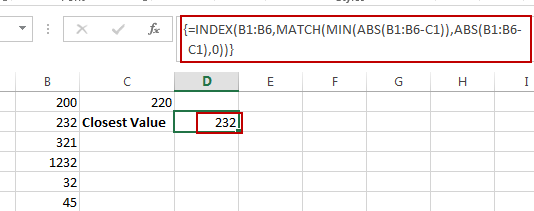## Find Closest Smaller Value

If you want to find the closest smaller value from a range of cells in excel, you need to use another formula based on the LARGE function and the COUNTIF function. Just like this:

`=LARGE(\$B\$1:\$B\$6,COUNTIF(\$B\$1:\$B\$6,">"&C1)+1)`

Type this formula into a blank cell, and then press Enter key. and the closest smallest value will be retrieved from the given range of cells.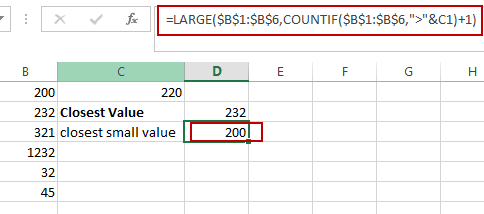## Find Closest Largest Value

If you want to find the closest largest value from a range of cells in excel, you need to use another formula based on the SMALL function and  the COUNTIF function. Just like this:

`=SMALL(\$B\$1:\$B\$6,COUNTIF(\$B\$1:\$B\$6,”<”&C1)+1)`

Type this formula into a blank cell, and then press Enter key. and the closest largest value will be retrieved from the given range of cells.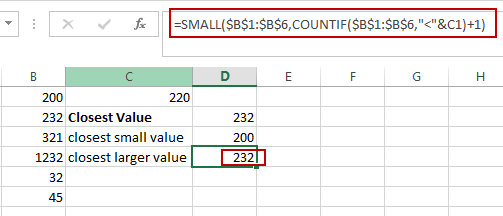### Related Functions

• Excel COUNTIF function
The Excel COUNTIF function will count the number of cells in a range that meet a given criteria. This function can be used to count the different kinds of cells with number, date, text values, blank, non-blanks, or containing specific characters.etc.= COUNTIF (range, criteria)…
• Excel INDEX function
The Excel INDEX function returns a value from a table based on the index (row number and column number)The INDEX function is a build-in function in Microsoft Excel and it is categorized as a Lookup and Reference Function.The syntax of the INDEX function is as below:= INDEX (array, row_num,[column_num])…
• Excel MATCH function
The Excel MATCH function search a value in an array and returns the position of that item.The syntax of the MATCH function is as below:= MATCH  (lookup_value, lookup_array, [match_type])….
• Excel SMALL function
The Excel SMALL function returns the smallest numeric value from the numbers that you provided. Or returns the smallest value in the array.The syntax of the SMALL function is as below:=SMALL(array,nth) …
• Excel MIN function
The Excel MIN function returns the smallest numeric value from the numbers that you provided. Or returns the smallest value in the array.The MIN function is a build-in function in Microsoft Excel and it is categorized as a Statistical Function.The syntax of the MIN function is as below:= MIN(num1,[num2,…numn])….
• Excel LARGE function
The Excel LARGE function returns the largest numeric value from the numbers that you provided. Or returns the largest value in the array. The syntax of the LARGE function is as below:= LARGE (array,nth)…
• Excel ABS Function
The Excel ABS function returns the absolute value of a number.The ABS function is a build-in function in Microsoft Excel and it is categorized as a Math and Trigonometry Function.The syntax of the ABS function is as below:=ABS (number)…

## Find the Largest Value Based on Multiple Criteria

This post will guide you how to find the largest value based on one criteria in excel. How do I return the largest value based on multiple criteria in excel. How to find and return the largest value based on one criteria and multiple criteria with formula in excel.

## Find Largest Value with multiple Criteria

Assuming that you have a list of data that you want to find the largest value based on the product “excel” and the sales region “east”. You can create a new excel formula based on the SUMPRODUCT function and the LARGE function, just using the following formula:

`=SUMPRODUCT(LARGE((\$A\$2:\$A\$6="excel")*(\$B\$2:\$B\$6="east")*(\$C\$2:\$C\$6),1))`

You just need to type this formula into the formula box of cell D2, then press Enter key in your keyboard.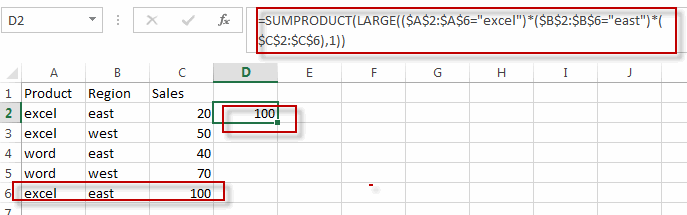This formula will find the first largest value based on the multiple criteria. And if you want to find the second largest value based on multiple criteria, just need to change “1” to “2”, just like this:

`=SUMPRODUCT(LARGE((\$A\$2:\$A\$6="excel")*(\$B\$2:\$B\$6="east")*(\$C\$2:\$C\$6),2))`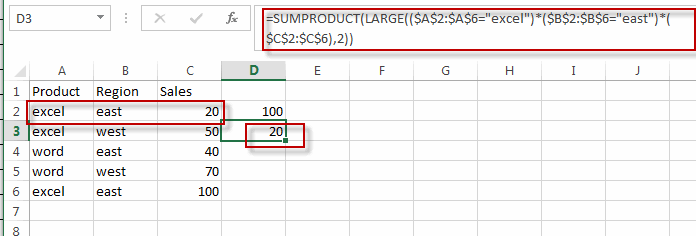## Find Largest Value with Only One Criteria

If you just want to find largest value with only one criteria, for example, you want to find the largest sales value based on the product “word”, then you can create a formula based on the LARGE function and the IF function. Just write down the following array formula as follows:

`=LARGE(IF(A2:A6="word",C2:C6),1)`

When you type this formula into the formula box of Cell D2, then press Ctrl +shift+ Enter keys together in your keyboard. Then the formula will become an array formula.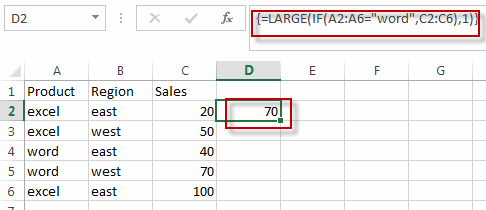This formula will get the first largest value based one criteria, and if you want to find the second or nth largest value based one criteria, you can change “1” to 2 or other number.

`=LARGE(IF(A2:A6="word",C2:C6),2)`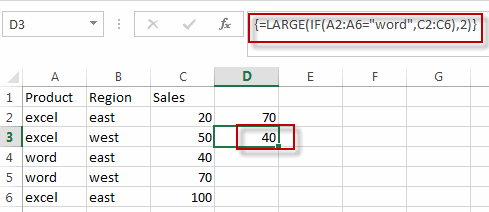### Related Functions

• Excel SUMPRODUCT function
The Excel SUMPRODUCT function multiplies corresponding components in the given one or more arrays or ranges, and returns the sum of those products.The syntax of the SUMPRODUCT function is as below:= SUMPRODUCT (array1,[array2],…)…
• Excel LARGE function
The Excel LARGE function returns the largest numeric value from the numbers that you provided. Or returns the largest value in the array. The syntax of the LARGE function is as below:= LARGE (array,nth)…
• Excel IF function
The Excel IF function perform a logical test to return one value if the condition is TRUE and return another value if the condition is FALSE. The IF function is a build-in function in Microsoft Excel and it is categorized as a Logical Function.The syntax of the IF function is as below:= IF (condition, [true_value], [false_value])….

## Excel LARGE Function

This post will guide you how to use Excel LARGE function with syntax and examples in Microsoft excel.

## Description

The Excel LARGE function returns the largest numeric value from the numbers that you provided. Or returns the largest value in the array. It also can be used to get the nth largest value from a range of cells or an array.

For example, the LARGE function can find the first, second, third or nth smallest value in a list or an array.

The LARGE function is a build-in function in Microsoft Excel and it is categorized as a Statistical Function.

The LARGE function is available in Excel 2016, excel 2013, excel 2010, excel 2007, excel 2003, excel XP, excel 2000, excel 2011 for Mac.

## Syntax

The syntax of the LARGE function is as below:

`= LARGE (array,nth)`

Where the LARGE function arguments are:

• array -This is a required argument.  An Array or a range of cells that contains numeric values from which you want to get the nth largest value.
• nth – This is a required argument.  The position of the number that you want to return

Note:

• If the first argument is empty, then the LARGE function returns #NUM! Error.
• If nth is less than or equal to 0 or if nth is greater than the number of values in array, the LARGE function returns the #NUM! Error.
• If nth is the number of values in array, then LARGE (array,1) returns the largest value, and LARGE(array,n) returns the smallest value.

## Excel LARGE Function Examples

The below examples will show you how to use Excel LARGE Function to get the largest value form the numbers in a range.

#1 To get the largest value from a range B1:B4, just using the following excel formula:

`=LARGE(B1:B4,1)`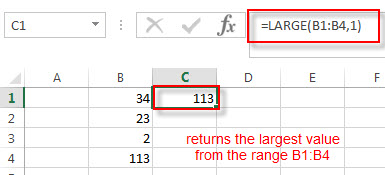The above formula returns the largest number from a range B1:B4, it returns 113.

2# To get the second largest value from the Range B1:B4, you can use the following LARGE function:

`=LARGE(B1:B4,2)`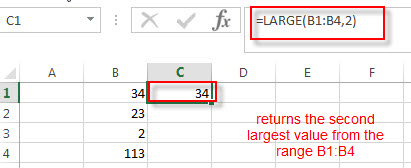The second argument nth in the above LARGE function is 2, so it will fetch the second largest function in the range B1:B4. It returns 34.

3# To get the largest value from the range B1:B4 that contains blank cells, enter into the following formula in the Cell C1:

`=LARGE(B1:B4,1)`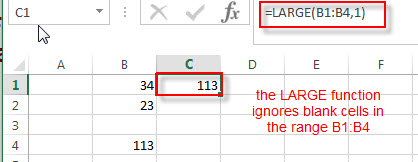The LARGE function will ignore blank cells in the Range. You will see that the Cell B3 is a blank cell, and it is ignored by the LARGE function. The largest value is still returned as 113.

4# To get the largest value from the range B1:B5 that contains text string, specific character and logical values. Using the following LARGE formula:

`=LARGE(B1:B5,1)`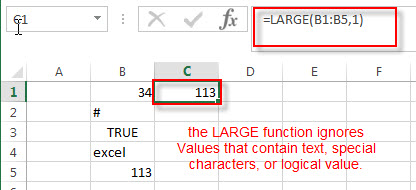The LARGE function will ignore values in the range B1:B5 that contain text string, specific character and logical value.

5# To get the largest value from the range B1:B5 that contain an error, using the below formula:

`=LARGE(B1:B5,1)`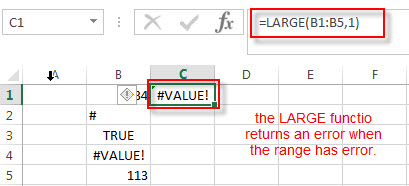### More Excel LARGE Function Examples

• Find the Largest Value Based on Multiple Criteria
Assuming that you have a list of data that you want to find the largest value based on the product “excel” and the sales region “east”. You can create a new excel formula based on the SUMPRODUCT function and the LARGE function..……
• Find Closest Value or Nearest Value in a Range
You need to use an excel array formula based on the INDEX function, the MATCH function, the MIN function and the ABS function to find Closest Value or Nearest Value in a Range in Excel…

## How to find the nth Largest Value

In the previous post, we talked that how to get the nth smallest value in a range and how to get the relative value of the same row of the nth smallest value in a range or array. And this post will guide you how to get the nth largest value in a range in excel. How to extract the relative value based on the position of the nth largest value in a single column.

## Find the nth Largest Value

To get the 1st, 2nd, 3rd, or nth largest value in a range (single column, or row), you can use the LARGE function.

For example:

Find the First Largest Value

You can use the following formula to get the first largest value in the range C2:C5:

`=LARGE(C2:C5,1)`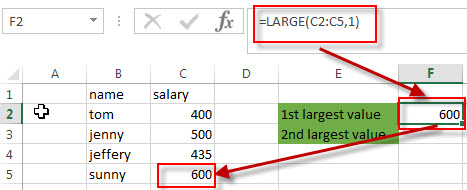Find the Second Largest Value

To get the second largest value in the range C2:C5, you can use the following LARGE function:

`=LARGE(C2:C5,2)`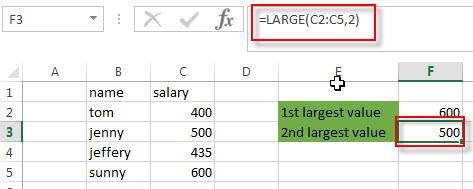Find the nth Largest Value

you just need to modify the second argument (nth) of the LARGE function to a numeric value that you want. Refer to the below formula:

`=LARGE(C2:C5,nth)`

## Get the Relative value

If you want to get the relative value of the same row of the nth largest value, you can use a combination with the LARGE function, the INDEX function and MATCH function. For example, to get the name value in the same relative row position of the second largest salary value in the range C2:C5, you can use the following excel formula:

`=INDEX(B2:B5,MATCH(LARGE(C2:C5,2),C2:C5,0))`

Let’s see how this formula works:

=LARGE(C2:C5,2)

As described above, This LARGE function returns the second largest value in the range C2:C5.

=MATCH(LARGE(C2:C5,2),C2:C5,0)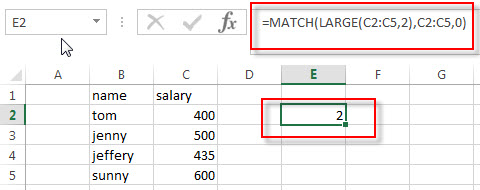The MATCH function will perform an exact searching in the range C2:C5, then returns the position number of the second largest value in the range C2:C5. It returns 2.

=INDEX(B2:B5,MATCH(LARGE(C2:C5,2),C2:C5,0))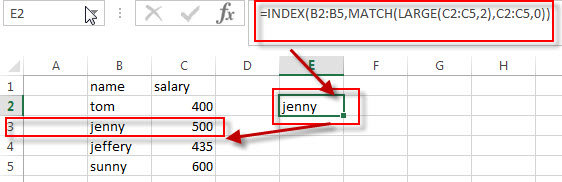The INDEX function extract the value based on the position number that returned by the above MATCH function, so it returns one value in the range B2:B5 based on the returned position. It returns “jenny”.

### Related Formulas

• Get nth Match with One Criteria using INDEX/MATCH
if you want to find the 2th occurrence of the member “jenny” in the range B2:B10 and extracts its relative bonus value in the range D2:D10, you can used the following array formula:=INDEX(D2:D10, SMALL(IF(B2:B10=”jenny”, ROW(B2:B10)-ROW(INDEX(B2:B10,1,1))+1),2))…
• Lookup the Value with Multiple Criteria
If you want to lookup the value with multiple criteria in a range, you can use a combination with the INDEX function and MATCH function to create an array formula.…
• Lookup the Next Largest Value
If you want to get the next largest value in another column, you can use a combination of the INDEX function and the MATCH function to create an excel formula..
• Find the nth Smallest Value
You can use the SMALL function to get the 1st, 2nd, 3rd, or nth smallest value in an array or range. Also you can use the SMALL function within the INDEX function to extract the relative value of the same row…

### Related Functions

• Excel INDEX function
The Excel INDEX function returns a value from a table based on the index (row number and column number)The INDEX function is a build-in function in Microsoft Excel and it is categorized as a Lookup and Reference Function.The syntax of the INDEX function is as below:= INDEX (array, row_num,[column_num])…
• Excel MATCH function
The Excel MATCH function search a value in an array and returns the position of that item.The syntax of the MATCH function is as below:= MATCH  (lookup_value, lookup_array, [match_type])….
• Excel LARGE function
The Excel LARGE function returns the largest numeric value from the numbers that you provided. Or returns the largest value in the array. The syntax of the LARGE function is as below:= LARGE (array,nth)…